# Modelling of Free Oscillations of Mass Spring System

March 25, 2022

By  Shubham

lang: en_US

# Modelling of Free Oscillations of a Mass-Spring System

## Introduction

Linear ordinary differential equations have critical applications in mechanical systems and electrical systems. In this blog, we will try to model and solve a simple mechanical system that will consist of a mass suspended on an elastic spring, also known as a "mass-spring system". The blog is in two parts. In the first part, we will start by setting up our mass-spring system with ideal conditions, i.e., having undamped motion. While in the second part, we will make our model realistic by introducing damping to our system. We will also discuss the three cases of damping.

## Setting up the Mass-Spring Model

Start by considering a mass suspended on an ordinary coil spring that resists compression and extension. The spring is suspended from a fixed support.Mass spring system

Assuming that we pull down the mass by an amount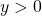, then, according to Hooke's law, there will be a spring force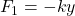.

Here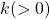is the spring constant. The negative sign indicates that the direction of forceis upward. There is also an additional force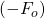in the spring. However,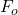does not affect the motion because it is in equilibrium with the weight of the ball, i.e.,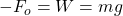The motion of this mass-spring system can be determined by Newton's second law of motion,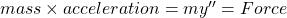.

## Forming the ODE of Undamped System

For now, we will consider that the damping of our system is minimal and, therefore, can be neglected. If we consider the motion of the system over a short time, then Newton's law gives the model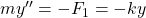; thus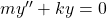The above equation is a homogeneous linear ODE and can be solved to obtain the general solution;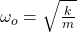This motion of the mass-spring system is called a harmonic oscillation and has the frequency.

## Implementing the Model of Mass-Spring System in MATLAB

Let's implement this model of simple harmonic motion in MATLAB and check the results. We will start with the following initialization condition:

% Initialization
W = 98;                              % weight of ball = 98nt
k = W/1.09;                        % spring constant
g = 9.8;                               % acceleration due to gravity
m = W/g;                            % mass of ball
w0 = sqrt(k/m);

MATLAB Codes!

We all came across the infamous mass-spring system during our physics classes. This time we will revisit it with the visualization power of MATLAB; Developed in MATLAB R2018a with Symbolic toolbox.

Now we will define the symbolic function for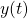and will try to solve the ODEfor the initial conditionsand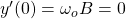. We will use the dsolve() function to solve the equation and get a particular solution.

Finally, we will plot the equations to get our graph of simple harmonic oscillations of our mass-spring system. Keep in mind that thehas a short-range (usually in seconds). Also, convert the values of the solution of ODE into a double type variable otherwise, MATLAB will give you an error.

On running the code, you should get a graph like the following.Undamped oscillations of Mass-spring system

## Introducing damping to the system

Now, lets introduce the damping force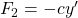to our system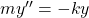whereis the damping constant. The ODE of this damped mass-spring system is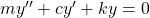. This damping force can be physically introduced by connecting the ball to a dashpot. Let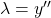, then the ODE can be represented as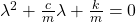. This equation is a quadratic equation and upon solving it, we get the roots as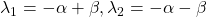. Here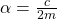and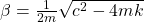. Depending upon the value of, there will be three cases of damping.

## Case 1: Over damping

If the damping constantis very large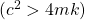, then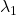and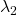become two distinct real roots and the general solution thus obtained for the ODE is,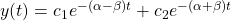Here, for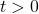, both the exponents have a negative value and hence both terms approach zero. Practically, the mass will be at rest at the static equilibrium position after a long time.

## Case 2: Critical damping

This case occurs when the roots of the equation are equal or we can say that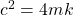. The general solution of the ODE isCritical damping is usually the case between nonoscillatory motions and oscillations.

## Case 3: Under damping

This case occurs when the damping constantis very small that is,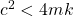. Here,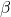is imaginary,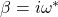Here,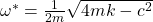The roots of the quadratic equation are complex conjugates and the corresponding general equation of the ODE is,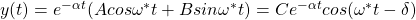This case represents the actual damped oscillations.

## Implementing the damped model of mass-spring system

We will start by introducing the damping constants to the initialization condition.

We will also introduce the new differential equations due to the three damping constants. Also, change the required lines that are used for solving the differential equations. Again, we are using the dsolve() function to solve the ODEs.

Now, just plot the graphs of these equations. This time we will also turn on legends so that we can differentiate between the different graphs and check which one belongs to over damping, critical damping and under damping.

Now, lets run the code and check our results.Damped oscillations of a Mass-spring System

Unlike the undamped mass-spring system, here, the system comes to rest after some time has passed.

## Summary

We have created an ideal mass-spring system with an ODE for our undamped system. We also implemented our system in MATLAB and got the desired results. We also introduced damping to this system. We discussed the three damping cases, i.e., overdamping, critical damping, and under damping, and checked its effects on the system using MATLAB.

## Conclusion

Mass-spring systems are the fundamental bodies used in modelling and solving certain engineering problems related to designing certain structures. Some of these problems are understanding the working of pendulums, designing the suspension systems of vehicles, analyzing the building response to an earthquake. The damped mass-spring systems are also employed to study certain designs' rigid body dynamics.

Ready to take your MATLAB skills to the next level? Look no further! At MATLAB Helper, we've got you covered. From free community support to expert help and training, we've got all the resources you need to become a pro in no time. If you have any questions or queries, don't hesitate to reach out to us. Simply post a comment below or send us an email at [email protected].

And don't forget to connect with us on LinkedIn, Facebook, and Subscribe to our YouTube Channel! We're always sharing helpful tips and updates, so you can stay up-to-date on everything related to MATLAB. Plus, if you spot any bugs or errors on our website, just let us know and we'll make sure to fix it ASAP.

Ready to get started? Book your expert help with Research Assistance plan today and get personalized assistance tailored to your needs. Or, if you're looking for more comprehensive training, join one of our training modules and get hands-on experience with the latest techniques and technologies. The choice is yours – start learning and growing with MATLAB Helper today!

Education is our future. MATLAB is our feature. Happy MATLABing!

#### Shubham

I have done my bachelor degree in electronics from University of Delhi. I like to tinker with the hardware to understand how it actually works.

#### MATLAB Helper ®

Use Website Chat or WhatsApp at +91-8104622179